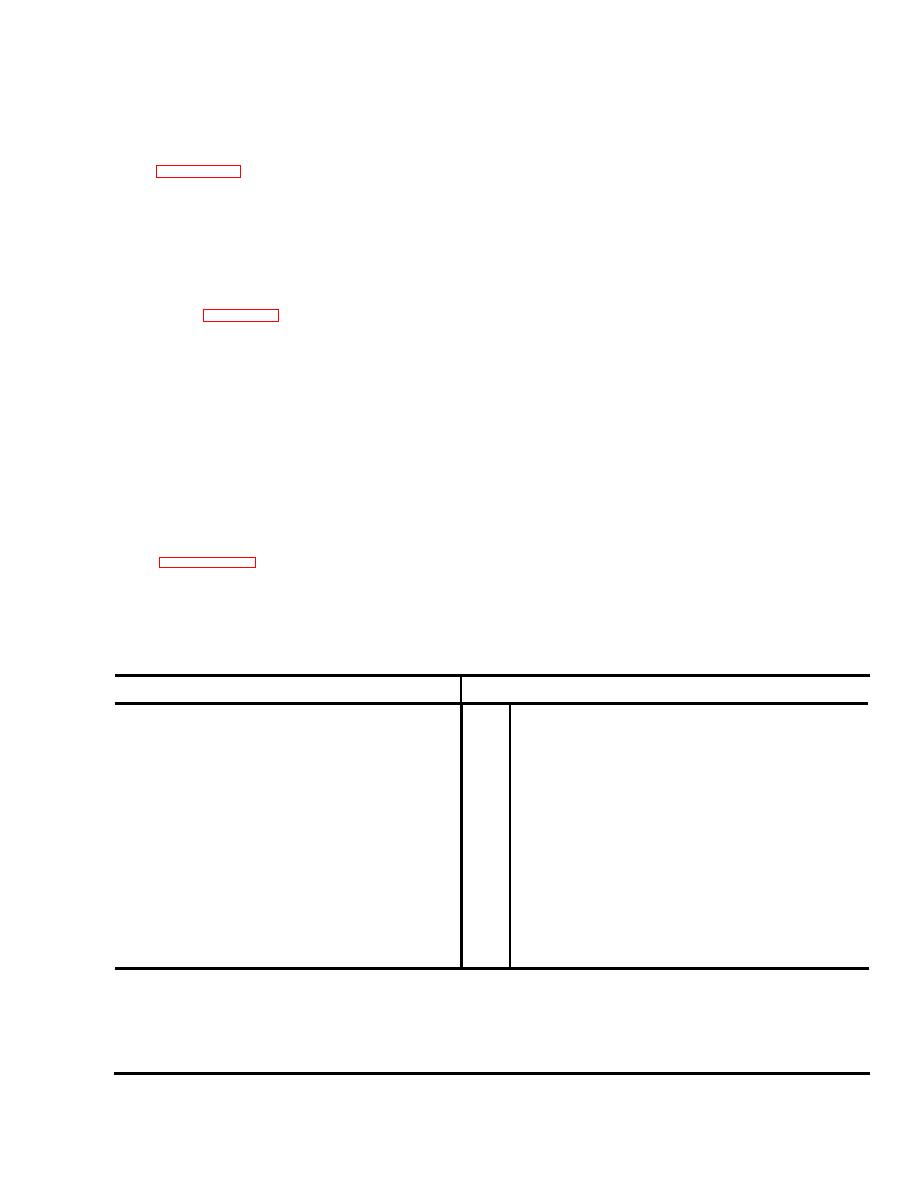Click here to make tpub.com your Home Page Page Title: Table 2-9. EQUATION SUMMARY Back | Up | NextWeb www.tpub.comHome

Information Categories
Aerographer
Automotive
Aviation
Construction
Diving
Draftsman
Engineering
Electronics
Food and Cooking
Logistics
Math
Medical
Music
Nuclear Fundamentals
Photography
ReligionTM
11-5820-919-40-1
by 200.
The  2,000,000  pulses  will
plus 4.91 MHz is 6.91 MHz, and
29.9999 MHz plus 4.91 MHz is 34.90
be  divided  to  10,000  pulses,  which
MHz .
corresponds  to  10  kHz.
This  will
match  the  10-kHz  Standard  and  the
Table  2-7  indicates  that  the  pre-
5.
VCO  tune  voltage  will  remain
scaler will pulse at a 1:11 ratio
constant.
nine times and then pulse at a
1:10  ratio  the  remaining  times
7.
The  approximate  VCO  tune  voltage
before it is reset.
The  high
can  be  determined  from  the  slope
frequency  loop  filter  will  send
formula
one reset pulse back to the
g
=
mP+b
prescaler  for  every  200  pulses
(see  table  2-8,  number  of  divi-
where
sions)  it  receives  from  the  pre-
g = the VCO tune voltage
scaler.
This  means  that  the  pre-
scaler  will  pulse  1:11  nine  times
m = 6.4/28.,000
. 2.29  X 1 0-4
and 1:10 191 times.
So, for the
first  2009  pulses  which  enter  the
P=
frequency
kHz
=
15,184.3
prescaler,  200  pulses  will  reach
=
the  high  frequency  loop  filter.
b
+2.13v
This  is  repeated  for  the  remainder
In this case, the
VCO
tune
voltage
of  the  20,090,000  pulses  (corre-
is +5.6v.
sponding  to  20,090,0  kHz)  until
2,000,000  pulses  reach  the  high
8.
Since the VCO tune voltage is con-
frequency  loop  filter.
stant , the  voltage  controlled  oscil-
lator  will  continue  to  oscillate
Table  2-8  indicates  that  the
6.
at 15,164.3 kHz, which is the LO
divide  counter  inside  the  high
frequency  loop  filter  will  divide
output .
EQUATION SUMMARY
Formula
Pin
Component
P kHz + 75,000 kHz = a
VCO, U3, LO
34
1.
S6 (.1) kHz + S5 kHz + 90.0 kHz = b
Low
Frequency
29
2.
Phase  Lock,  U5
b kHz + 70,000 = C
Mixer
U6
1
3.
a kHz - C k H z = d k H z
19
4.
VCO,
U3,
mixer
= P MHz + 4.91 MHz
(d kHZ - (S4
+
1)
10
kHz)/10
Prescaler,
U4
39
5.
(S1 (100) + S2 (10) + S3) + 49
High  Frequency Loop
Filter
U2,
6.
...
divide  number
g = mP + b = VCO tune voltage
High  Frequency Loop
Filter
U2,
18
7.
b =  +2.13
VCO  tune  voltage
m = 2.29  X 1 0-4
Where
P is the control panel
S3
is
the
100-kHz digit (1)
frequency  in  kHz.
S4
is
the
10-kHz digit
(8)
S1  is  the  10-MHZ  digit
(1)
S5
is
the
1-kHz digit
(4)
S6
is
the
100-HZ, digit
S2 is the 1-MHz digit
(5)
(3)Integrated Publishing, Inc. - A (SDVOSB) Service Disabled Veteran Owned Small Business